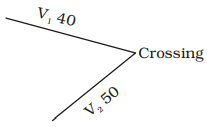## Speed, Time and Distance

#### Speed, Time and Distance

1. A speed of 45 km per hour is the same as
1. 12.5 metre/seccond
2. 13 metre/seccond
3. 15 metre/seccond
4. 12 metre/seccond

1. Speed = 45 kmph

 =45 × 1000metre/second 60 × 60

 =45 × 5metre/second 18

= 12.5 metre/second

##### Correct Option: A

Speed = 45 kmph

 =45 × 1000metre/second 60 × 60

 =45 × 5metre/second 18

= 12.5 metre/second

1. The distance between place A and B is 999 km. An express train leaves place A at 6 am and runs at a speed of 55.5 km/hr. The train stops on the way for 1 hour 20 minutes. It reaches B at
1. 1.20 am
2. 12 pm
3. 6 pm
4. 11 pm

1. Time taken in covering 999 km

 = 999 = 18 hours 55.5

∴  Required time = 18 hours + 1 hour 20 minutes
= 19 hours 20 minutes
i.e. 1 : 20 am

##### Correct Option: A

Time taken in covering 999 km

 = 999 = 18 hours 55.5

∴  Required time = 18 hours + 1 hour 20 minutes
= 19 hours 20 minutes
i.e. 1 : 20 am

1. Two cars are moving with speed v1, v2 towards a crossing along two roads. If their distance from the crossing be 40 metres and 50 metres at an instant of time then they do not collide if their speed are such that
1. v1 : v2 = 16 : 25
2. v1 : v2 ≠ 4 : 5
3. v1 : v2 ≠ 5 : 4
4. v1 : v2 = 25 : 16

1.Let time taken be equal

 i.e., 40 = 50 ,   then V1 V2

they will collide i.e. cars will reach at the same time.
 ∴ V1 ≠ 40 = 4 V2 50 5

##### Correct Option: BLet time taken be equal

 i.e., 40 = 50 ,   then V1 V2

they will collide i.e. cars will reach at the same time.
 ∴ V1 ≠ 40 = 4 V2 50 5

1. A, B, C walk 1 km in 5 minutes, 8 minutes and 10 minutes respectively. C starts walking from a point, at a certain time, B starts from the same point 1 minutes later and A starts from the same point 2 minutes later than C. Then A meets B and C after
1.  5 min, 2 min 3
2. 1 min, 2 min
3. 2 min, 3 min
4.  4 min, 3 min 3

1.  A’s speed = 1000 = 200 m/minute 5

 B’s speed = 1000 = 125 m/minute 8

 C’s speed = 1000 = 100 m/minute 10

Distance covered by C in 2 minutes = 200 metre
Distance covered by B in 1 minute = 125 metre
Relative speed of A with respect to C = 100 metre
 ∴  Time = 200 = 2 minutes 100

Relative speed of A with respect ot B = 75 metre
 ∴  Time = 125 = 5 minutes 75 3

##### Correct Option: A

 A’s speed = 1000 = 200 m/minute 5

 B’s speed = 1000 = 125 m/minute 8

 C’s speed = 1000 = 100 m/minute 10

Distance covered by C in 2 minutes = 200 metre
Distance covered by B in 1 minute = 125 metre
Relative speed of A with respect to C = 100 metre
 ∴  Time = 200 = 2 minutes 100

Relative speed of A with respect ot B = 75 metre
 ∴  Time = 125 = 5 minutes 75 3

1.  Ram travelled 1200 km by air which formed 2 of his trip. 5
He travelled one-third of the trip by car and the rest by train. The distance (in km) travelled by train was
1. 480
2. 800
3. 1600
4. 1800

1. Total distance of trip

 = 1200 × 5 = 3000 km 2

Part of journey covered by train
 = 1 − 2 − 1 = 15 − 6 − 5 = 4 5 3 15 15

 ∴  Distance covered by train = 3000 × 4 15

= 800 km

##### Correct Option: B

Total distance of trip

 = 1200 × 5 = 3000 km 2

Part of journey covered by train
 = 1 − 2 − 1 = 15 − 6 − 5 = 4 5 3 15 15

 ∴  Distance covered by train = 3000 × 4 15

= 800 km